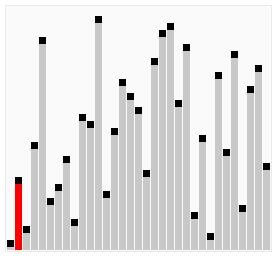# Python Example – Write a Python program to sort a list of elements using Cocktail shaker sort

Hits: 21# (Python Example for Citizen Data Scientist & Business Analyst)

Write a Python program to sort a list of elements using Cocktail shaker sort.

From Wikipedia, Cocktail shaker sort, also known as bidirectional bubble sort, cocktail sort, shaker sort (which can also refer to a variant of selection sort), ripple sort, shuffle sort, or shuttle sort, is a variation of bubble sort that is both a stable sorting algorithm and a comparison sort. The algorithm differs from a bubble sort in that it sorts in both directions on each pass through the list. This sorting algorithm is only marginally more difficult to implement than a bubble sort, and solves the problem of turtles in bubble sorts. It provides only marginal performance improvements, and does not improve asymptotic performance; like the bubble sort, it is not of practical interest (insertion sort is preferred for simple sorts), though it finds some use in education.

Visualization of shaker sort:Sample Solution:

Python Code:

``````
def cocktail_shaker_sort(nums):
for i in range(len(nums)-1, 0, -1):
is_swapped = False

for j in range(i, 0, -1):
if nums[j] < nums[j-1]:
nums[j], nums[j-1] = nums[j-1], nums[j]
is_swapped = True

for j in range(i):
if nums[j] > nums[j+1]:
nums[j], nums[j+1] = nums[j+1], nums[j]
is_swapped = True

if not is_swapped:
return nums

num1 = input('Input comma separated numbers:n').strip()
nums = [int(item) for item in num1.split(',')]
print(cocktail_shaker_sort(nums))
``````

Sample Output:

```Input comma separated numbers:
15, 37, 69, 26, 78
[15, 26, 37, 69, 78]```

## Write a Python program to sort a list of elements using Cocktail shaker sort

#### Free Machine Learning & Data Science Coding Tutorials in Python & R for Beginners. Subscribe @ Western Australian Center for Applied Machine Learning & Data Science.```